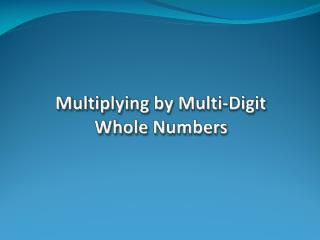Download PresentationMultiplying by Multi-Digit Whole Numbers

Multiplying by Multi-Digit Whole Numbers - PowerPoint PPT Presentation

Multiplying by Multi-Digit Whole Numbers. Multiplying by Multi-Digit Whole Numbers. Definitions: Factors - Are the individual numbers that are multiplied together. Product - Is the answer when two or more numbers are multiplied together . 12 x 9 108. Factors. Product.I am the owner, or an agent authorized to act on behalf of the owner, of the copyrighted work described.
Download PresentationMultiplying by Multi-Digit Whole Numbers

Download Policy: Content on the Website is provided to you AS IS for your information and personal use and may not be sold / licensed / shared on other websites without getting consent from its author.While downloading, if for some reason you are not able to download a presentation, the publisher may have deleted the file from their server.

- - - - - - - - - - - - - - - - - - - - - - - - - - E N D - - - - - - - - - - - - - - - - - - - - - - - - - -
Presentation Transcript
1. Multiplying by Multi-Digit Whole Numbers

2. Multiplying by Multi-Digit Whole Numbers Definitions: Factors -Are the individual numbers that are multiplied together. Product -Is the answer when two or more numbers are multiplied together. 12 x 9 108 Factors Product

3. Multiplying by Two-Digit Whole Numbers Without Regrouping Example 1: 23 x 13 69 + 230 299 Multiply the 3 in “13” by each digit in 23. Put a “0” in the ones place and multiply the 1 in “13” by each digit in 23. Add all of the partial products to get your final answer.

4. Multiplying by Two-Digit Whole Numbers With Regrouping Example 2: 993 x 68 7944 + 59580 67,524 Multiply the 8 in “68” by each digit in 993. Put a “0” in the ones place and multiply the 6 in “68” by each digit in 993. Add all of the partial products to get your final answer.

5. Multiplying by Multi-Digit Whole Numbers With Regrouping Example 3: 712 x 564 2848 42720 +356000 401,568 Multiply the 4 in “564” by each digit in 712. Put a “0” in the ones place and multiply the 6 in “564” by each digit in 712. Put a “0” in the ones place and in the tens place and multiply the 5 in “564” by each digit in 712 . Add all of the partial products to get your final answer.

6. Multiplying by Three-Digit Whole Numbers Try these: 1.) 12 2.) 671 x 32x 54 Challenge: 3.) 751 4.) 2,213 x 234x 639

7. Multiplying by Multi-Digit Whole Numbers Real-Life Application Try this one: Mark makes \$663 per week. If he works 49 weeks this year, how much will he make for the entire year? Show all work.